## Example Questions

### Example Question #1 : How To Find The Height Of A Right Triangle

Given a right triangle with a leg length ofand a hypotenuse length of, what is the height of the triangle?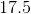Explanation:

1. Use the pythagorean theorem with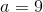and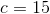: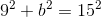2. Solve for: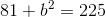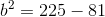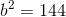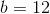(Notice that this right triangle is also a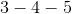triangle.)

### Example Question #2 : How To Find The Height Of A Right Triangle

A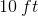ladder is leaning against a wall. If the bottom of the ladder touches the ground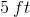from the base of the wall, approximately (to the nearest whole number) how far is the top of the ladder from the base of the wall?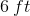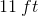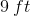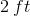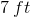Explanation:

To answer this, we first need to understand that we are trying to find the leg of a right triangle. The ladder, when leaned against the wall, forms a right triangle where the ladder is the hypotenuse, the wall is one leg, and the ground between the ladder and the wall is the other leg.

To find how high up the ladder touches the wall, we use the Pythagorean Theorem, which is: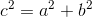is the length of the hypotenuse (the ladder itself),is the distance of the ladder base from the wall, andis how high the ladder touches on the wall.

We then rearrange to solve for one of the legs by subtracting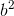from both sides.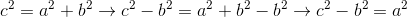We can now plug in our values of 10 for the hypotenuse and one of our legs (in this case,)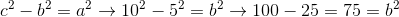We can then take the square root of this equation to get an answer for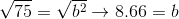The question asked us to approximate, so we must round to the nearest whole number. To do this, we round a number up one place if the last digit is a 5, 6, 7, 8, or 9, and we round it down if the last digit is a 1, 2, 3, or 4. Therefore: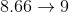Therefore, our answer is.

### Example Question #3 : How To Find The Height Of A Right Triangle

Right triangle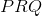has sides that are integers, and an area of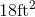. Which of the following could not be the height of?Explanation:

The formula for the area of a triangle is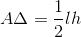, whereis the length andis the height of the triangle. In this case, we know that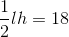, which means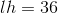. Since we are told that the sides are integers, this means that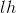must consist of two factors of. Sinceis the only number choice not a factor of, it is the only answer that could not meet the requirements.

### Example Question #4 : How To Find The Height Of A Right Triangle

Find the height of a right triangle given a base ofand hypotenuse of.To solve, simply realize this is one of our special right triangles,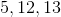. Thus, our answer is. If you forgot this, you can use the Pythagorean Theorem.